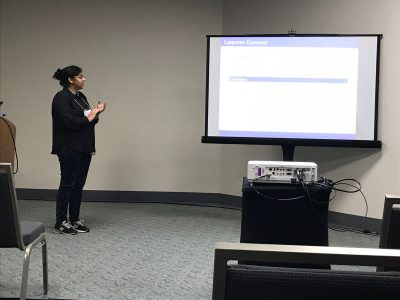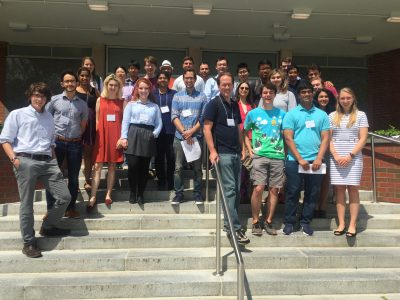# Fractional Gaussian fields on surfaces and graphs

June 24, 2022

Group Members: Tyler Campos, Andrew Gannon, Benjamin Hanzsek-Brill, Connor Marrs, Alexander Neuschotz, Trent Rabe and Ethan Winters.

Overview: We will study and simulate on computers the fractional Gaussian fields and their discretizations on surfaces like the two-dimensional sphere or two-dimensional torus. The study of the maxima of those processes will be done and conjectures formulated concerning limit laws. Particular attention will be paid to log-correlated fields (the so-called Gaussian free field).

July 9, 2020

preprint

# Box-ball systems and RSK tableaux

July 8, 2020

`Sém. Lothar. Combin.  85B  (2021), Art. 14, 12 pp.`

Proceedings of the 33rd Conference on Formal Power
Series and Algebraic Combinatorics

Ben Drucker, Eli Garcia, and Rose Silver

A box-ball system is a collection of discrete time states representing a permutation,
on which there is an action called a BBS move. After a finite number of BBS moves
the system decomposes into a collection of soliton states; these are weakly
increasing and invariant under BBS moves. The students proved that when this
collection of soliton states is a Young tableau or coincides with a partition of a type
described by Robinson-Schensted (RS), then it is an RS insertion tableau. They also
studied the number of steps required to reach this state.

# Hedging by Sequential Regression in Generalized Discrete Models and the Follmer-Schweizer decomposition

August 3, 2019## Overview

In practice, financial models are not exact — as in any field, modeling based on real data introduces some degree of error. However, we must consider the effect error has on the calculations and assumptions we make on the model.  In complete markets, optimal hedging strategies can be found for derivative securities; for example, the recursive hedging formula introduced in Steven Shreve’s “Stochastic Calculus for Finance I” gives an exact expression in the binomial asset model, and as a result the unique arbitrage-free price can be computed at any time for any derivative security.

In incomplete markets this cannot be accomplished; one possibility for computing optimal hedging strategies is the method of sequential regression.  We considered this in discrete-time; in the (complete) binomial model we showed that the strategy of sequential regression introduced by Follmer and Schweizer  is equivalent to Shreve’s recursive hedging formula, and in the (incomplete) trinomial model we both explicitly computed the optimal hedging strategy predicted by the Follmer-Schweizer decomposition and we showed that the strategy is stable under small perturbations.

# Geodesic Interpolation on the Sierpinski Gasket

July 9, 2018

## Overview

Geodesics (shortest paths) on manifolds such as planes and spheres are well understood.  Geodesics on fractal sets such as the Sierpinski Triangle are much more complicated.  We begin by constructing algorithms for building shortest paths and provide explicit formulas for computing their lengths.  We then turn to the question of interpolation along geodesics—given two subsets of the Sierpinski Triangle, we “slide” points in one set along geodesics to the other set.  We construct a measure along the interpolated sets which formalizes a notion of the interpolation of a distribution of mass, and we prove interesting self-similarity relations about this measure.

# Math UConn REU at JMM 2018

January 6, 2018

Two of our REU (2017 Stochastics) participants, Raji Majumdar and Anthony Sisti, will be presenting posters Applications of Multiplicative LLN and CLT for Random Matrices and Black Scholes using the Central Limit Theorem on Friday, January 12 at the MAA Student Poster Session, and both of them will be giving talks on Saturday, January 13 at the AMS Contributed Paper Session on Research in Applied Mathematics by Undergraduate and Post-Baccalaureate Students.

Their travel to the 2018 JMM has been made possible with the support of the MAA and UConn’s OUR travel grants.# 5th Northeast Mathematics Undergraduate Research Mini-Symposium

August 17, 2017

### University of Connecticut, August 3rd, 2017

#### Participating Schools: Amherst, Smith, UConn and UMass

5th Mini-Symposium full program (2017)July 28, 2017

## Overview

Laplacians have been well studied on post-critically finite (PCF) fractals. However, less is known about gradients on such fractals. Building on work by Teplyaev, we generalize results regarding the existence and continuity of the gradient on the standard Sierpinski Gasket to higher dimensional Sierpinski Gaskets. In particular, we find that, for functions with a continuous Laplacian, the gradient must be defined almost everywhere, and specify a set of points for which it is defined. Furthermore, we provide a counterexample on higher-dimensional Sierpinski gaskets where the Laplacian is continuous but the gradient is not defined everywhere. We conjecture that Hölder continuity of the Laplacian is a condition strong enough to guarantee that the gradient exists at each point.

# Multiplicative LLN and CLT and their Applications

July 25, 2017

## Overview

We study the Law of Large Numbers (LLN) and and Central Limit Theorems (CLT) for products of random matrices. The limit of the multiplicative LLN is called the Lyapunov exponent. We perturb the random matrices with a parameter and we look to find the dependence of the the Lyapunov exponent on this parameter. We also study the variance related to the multiplicative CLT. We prove and conjecture asymptotics of various parameter dependent plots.

## Presentations:

Raji Majumdar and Anthony Sisti, will present posters Applications of Multiplicative LLN and CLT for Random Matrices and Black Scholes using the Central Limit Theorem on Friday, January 12 at the MAA Student Poster Session, and give talks on Saturday, January 13 at the AMS Contributed Paper Session on Research in Applied Mathematics by Undergraduate and Post-Baccalaureate Students.